# Tabular Integration (The tabular method) Examples |Where to use tabular integration by parts method|

Home  > Math Article > Tabular Integration Method

Page Contents

## What is Tabular Integration method and when to use?

Tabular integration is a short method for integration to solve the integral problem quickly instead of using the lengthy and tedious process of integration by parts traditional method.

The advantage of the tabular integration method is that it can save huge time in solving the problem and gives the solution fairly accurate than the integration by parts method. This tabular method is fairly quick to memorize and easy to use.

This method is also called the DI method of Integration by parts.

## When can I use the Tabular integration method?

The tabular integration method can be applied to any function which is the product of two expressions, where one of the expressions can be differentiated until it gets zero, and another expression can be integrated simultaneously multiple times.

Suppose a function, f(x) = m(x).n(x), from given two expression, one of the expression, let’s take it m(x) should be differentiate multiple times until it reach to zero, and the another expression n(x) should be integrated simultaneously multiple times.

Note: Tabular method can also be used where neither of the expression differentiation goes to zero. Example – (∫e2x.sin3x dx) or (∫sin3x.cos4x dx), etc.

Take a look at where we can apply the tabular integration method.

• When Integrand is the product of Polynomial times and something that can be repeatedly integrated.  (x10.cos x dx)
• Integrand multiple of power function and an exponential function. (∫x².ex dx)
• Integrand multiple of an exponential and trigonometry function. (∫ex.sin x dx)
• Integrand multiple of power function and a trigonometry function. (∫x³.tan x dx)

## How to use or apply the Tabular integration method and its formulas?Let the understand by taking the example of the integral function

$\int _ { } ^ { } t ^ { 2 } \sin t dt$

Step 1 = First identify the polynomial function or the function which is differential. So, t² is a polynomial function and denote it as F(x)
F(x) = t²
Step 2 = Now take another product left in function which is integrable and denotes it as F(y)
F(y) = sint
Step 3 = Create a table and differentiate F(x) until it reaches 0 and simultaneously integrates F(y).
Step 4 = Now change the sign of every second term of the F(x) function to negative Or Add alternating “+” and “-” signs, starting with “+” on the derivatives side of the table.

### Tabular Integration Method

Now we have, F(x) = t2 and F(y) = sint

 F(x) Differentiate F(y) Integration t² sin t 2t -cos t 2 -sin t 0 cos t

Now we will add alternating “+” and “-” signs, starting with “+” on the derivatives side of the table.

 F(x) Differentiate F(y) Integration + t² sin t – 2t -cos t + 2 -sin t 0 cos t

Now Most important Steps in the tabular integration method to find the integral of a given function

1. Multiply F(x) with the first integration of F(y)
2. Multiply the first derivative of F(x) with the second integration of F(y)………and so on.So, $\int _ { } ^ { } t ^ { 2 } \sin t dt$ = t²(-cost) + (-2t)(-sint) + 2cost + C

= -t²cost + 2tsint + 2cost + C   Answer

## Tabular Integration Method for solving Definite Integral with limits problems

The definite integral is those integrals that have upper and lower limits or bounds or boundaries. In short, definite integral has start and end values.

Now, How to solve definite integral problems by the Tabular Integration rather than the traditional method and save our time.

### Example 1:Step 1 = First identify the polynomial function or the function which is differential. So, t³ is a polynomial function and denote it as F(x)
F(x) = t³
Step 2 = Now take another product left in function which is integrable and denotes it as F(y)
F(y) = sin t
Step 3 = Create a table and differentiate F(x) until you reach 0 and simultaneously integrate F(y).
Step 4 = Now change the sign of every second term of the F(x) function to negative Or add alternating “+” and “-” signs, starting with “+”:
Now we have, F(x) = t3 and F(y) = sint
 F(x) Derivative Function F(y) Integration Function (+)   t³ sin t (-)   3t² -cos t (+)   6t -sin t (-)   6 cos t 0 sin t

• Multiply F(x) with the first integration of F(y)
• Multiply the first derivative of F(x) with the second integration of F(y)………and so on.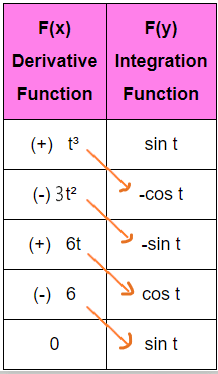$\int _ { 0 } ^ { \pi } t ^ { 3 } \sin t dt$  = t³( -cost ) $\Big| _ 0 ^ \pi$ – 3t² ( -sint ) $\Big| _ 0 ^ \pi$ + 6tcost $\Big| _ 0 ^ \pi$  – 6sint $\Big| _ 0 ^ \pi$

= -t³cost $\Big| _ 0 ^ \pi$  + 3t²sint $\Big| _ 0 ^ \pi$  + 6tcost $\Big| _ 0 ^ \pi$  – 6(sint)$\Big| _ 0 ^ \pi$

= [-π³cosπ – 0] + [3π².0 – 0] + [6πcosπ – o] + [-6(0 – 0]

= -π³(-1) + 6π(-1) + 0

So, Definite integral of the function $\int _ { 0 } ^ { \pi } t ^ { 3 } \sin t dt$ by tabular integration by parts method is π³ – 6π

### Example 2:Solution: Using same procedure, Make F(x) = t   and   F(y) = Cos2t

Now construct the table and take appropriate action to solve these definite integral problems by the tabular method

 F(x) Derivative Function F(y) Integration Function (+)   t Cos2t (-)   1 (1/2)Sin2t (+)   0 -(1/4)Cos2t

Follow these steps for further solving definite integral by the tabular method

1. Multiply F(x) with the first integration of F(y)
2. Multiply the first derivative of F(x) with the second integration of F(y)………and so on.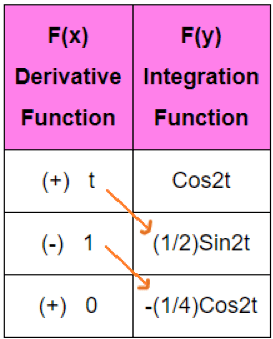$\int _ { 0 } ^ { \frac { \pi } { 4 } } t \cos2t dt$ = t/2Sin2t $\Big| _ 0 ^ \frac { \pi } { 4 }$ + 1/4Cos2t $\Big| _ 0 ^ \frac { \pi } { 4 }$

= $[ \frac { \pi } { 8 } \sin \frac { \pi } { 2 } – 0 ]$  + $\frac { 1 } { 4 } [ \cos \frac { \pi } { 2 } – \cos0 ]$

= $\frac { \pi } { 8 } + \frac { 1 } { 4 } ( 0 – 1 )$

= $\frac { \pi – 2 } { 8 }$   Answer

## Tabular Integration Examples with their Solutions

Let’s take a few more examples to understand the tabular integration method completely.

### Example 1:

Solving problems based on exponential and trigonometric functions using Tabular Integration.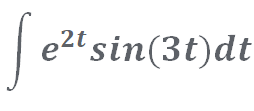Solution: Now you are thinking that there is no function in the above example that comes up 0 after derivating it endlessly. Then how can we use the tabular integration method here?

Don’t worry just follow the good approach.

Assume, F(x) = sin3t  and  F(y) = e2t

Construct tabular method table for integrating this problem

 F(x) Derivative Function F(y) Integration Function Sin(3t)    (+) e2t 3cos(3t)   (-) 1/2(e2t) -9sin(3t)   (+) 1/4(e2t)

1. Multiply F(x) with the first integration of F(y)
2. Multiply the first derivative of F(x) with the second integration of F(y).
3. Multiply the second derivative of F(x) with the second integration of F(y).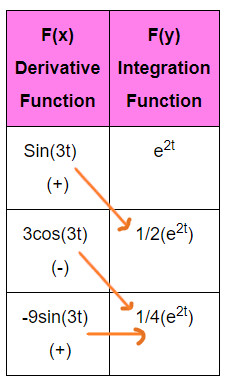So, $\int _ { } ^ { } e ^ { 2t } \sin3t dt$ = $\frac { 1 } { 2 }$ e2t sin3t – $\frac { 3 } { 4 }$ e2t cos3t – $\frac { 9 } { 4 }$ $\int _ { } ^ { } e ^ { 2t } \sin3t dt$

$\frac { 13 } { 4 }$ $\int _ { } ^ { } e ^ { 2t } \sin3t dt$ = $\frac { 1 } { 2 }$ e2t sin3t – $\frac { 3 } { 4 }$ e2t cos3t + C

$\int _ { } ^ { } e ^ { 2t } \sin3t dt$ = $\frac { 1 } { 13 }$ e2t  [ 2sin3t – 3cos3t ] + C   Answer

### Example 2:Solution: let’s give a shot at this integral problem by using the tabular integration method.

Look at the problem and choose a function that will give 0 value after derivating multiple times.

F(x) = t3 – 4t2 + 5t + 32  and   F(y) = sint

Construct the table to solve this problem by Tabular integration method

 F(x) Derivative function F(y) Integration function (+)     t3 -4t2 + 5t + 32 sint (-)       3t2 – 8t + 5 -cost (+)       6t – 8 -sint (-)           6 cost 0 sint

Use same steps

1. Multiply F(x) with the first integration of F(y)
2. Multiply the first derivative of F(x) with the second integration of F(y)………and so on.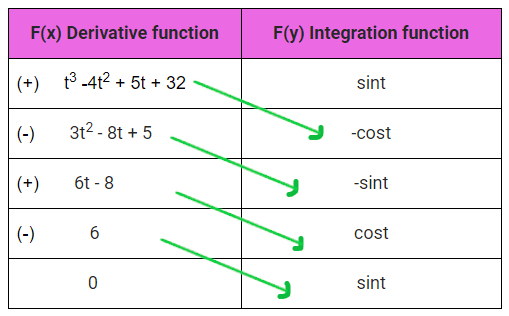So, we have  $\int _ { } ^ { } (t ^ { 3 } – 4t ^ { 2 } + 5t + 32)(sint) dt$  = -(t3 – 4t2 + 5t + 32)(cost) + (3t2 – 8t + 5)(sint) + (6t – 8)(cost) – 6sint + C

Simplify form = (3t2 8t 1)sin(t) + (t3 + 4t2 + t40)cos(t) + C     Answer

### Example 3:

Solving problems based on power and exponential function using Tabular integration.Solution: F(x) = t   and    F(y) = e-t

Construct the table to solve this integral problem by tabular integration method

 F(x) Derivative Function F(y) Integration Function (+) t5 e-t (-) 5t4 -e-t (+) 20t³ e-t (-) 60t² -e-t (+) 120t e-t (-) 120 -e-t (+) 0 e-t

Use same steps

1. Multiply F(x) with the first integration of F(y)
2. Multiply the first derivative of F(x) with the second integration of F(y)………and so on.$\int _ { } ^ { } t ^ { 5 } e ^ { – t } dt$ = t5(-e-t) – 5t4(e-t) + 20t3(-e-t) – 60t2(e-t) + 120t(-e-t) – 120(e-t) + C

$\int _ { } ^ { } t ^ { 5 } e ^ { – t } dt$ = -t5(e-t) – 5t4(e-t) – 20t3(e-t) – 60t2(e-t) – 120t(e-t) – 120(e-t) + C

= (t5 + 5t4 + 20t3 + 60t2 + 120t + 120)et + C    Answer

### Example 4:

Solving problem based on two trigonometric function using Tabular integration method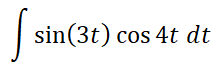Solution: We know, in the above problem, both function sin3t and cos4t will not get zero regardless of how much time you can differentiate them.

So, take, any of the functions as F(x) and another one as F(y) and apply the Tabular integration method.

So, I assume,  F(x) = Sin(3t)  and  F(y) = Cos(4t)                [∴You can choose whatever you want]

Now construct the table for solving this by tabular method

 F(x) Derivative Function F(y) Integration Function (+)   Sin(3t) Cos(4t) (-)   3Cos(3t) $\frac { 1 } { 4 }$ Sin(4t) (+)  -9Sin(3t) $– \frac { 1 } { 16 }$Cos(4t)

Use these steps solve this integral problem

1. Multiply F(x) with the first integration of F(y)
2. Multiply the first derivative of F(x) with the second integration of F(y).
3. Multiply the second derivative of F(x) with the second integration of F(y).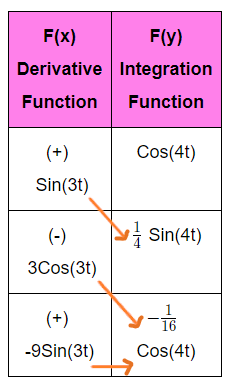$\int _ { } ^ { } \sin3t \cos4t dt$  = $\frac { 1 } { 4 }$ Sin(3t)Sin(4t) + $\frac { 3 } { 16 }$ Cos(3t)Cos(4t) + $\frac { 9 } { 16 }$ $\int _ { } ^ { } \sin3t \cos4t dt$

$\frac { 7 } { 16 } \int _ { } ^ { } \sin3t \cos4t dt$ = $\frac { 1 } { 4 }$ Sin(3t)Sin(4t) + $\frac { 3 } { 16 }$ Cos(3t)Cos(4t) + C

$\int _ { } ^ { } \sin3t \cos4tdt$ = $\frac { 16 } { 7 }$ [ $\frac { 1 } { 4 }$ Sin(3t)Sin(4t) + $\frac { 3 } { 16 }$ Cos(3t)Cos(4t) ] + C

= [ $\frac { 4 } { 7 }$ Sin(3t)Sin(4t) + $\frac { 3 } { 7 }$ Cos(3t)Cos(4t) ] + C   Answer

### Example 5:

Based on when you have only one function in the problem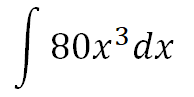Solution: We can solve this problem by assuming as other function 1 and then apply tabular integration method.

So, let F(x) = 80x³    and    F(y) = 1

Construct the table to solve this problem by tabular integration method

 F(x) Derivative Function F(y) Integration Function (+) 80x³ 1 (-)   240x² x (+)   480x x²/2 (-)   480 x³/6 (+)   0 x4/24

Use these steps to further solve this integral problem.

1. Multiply F(x) with the first integration of F(y)
2. Multiply the first derivative of F(x) with the second integration of F(y)………and so on.So, $\int _ { } ^ { } 80x ^ { 3 } dx$ = $80x ^ { 4 } – 240 \frac { x ^ { 4 } } { 2 } + 480 \frac { x ^ { 4 } } { 6 } – 480 \frac { x ^ { 4 } } { 24 }$

= $80x ^ { 4 } – 120x ^ { 4 } + 80x ^ { 4 } – 20x ^ { 4 }$

= $20x ^ { 4 } + C$ Answer

### Example 6: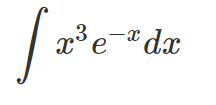Solution: Let F(x) = x³    and    F(y) = e-x

Construct the table to solve this problem by tabular integration method

 F(x) Derivative Function F(y) Integration Function (+) x³ e-x (-)   3x² – e-x (+)   6x e-x (-)   6 – e-x (+)   0 e-x

Use these steps to further solve this integral problem.

1. Multiply F(x) with the first integration of F(y)
2. Multiply the first derivative of F(x) with the second integration of F(y)………and so on.$\int _ { } ^ { } x ^ { 3 } e ^ { – x } dt$ = +x3(-e-x) – 3x2(e-x) + 6x(-e-x) – 6(e-x) + C

= – x3.e-x – 3x2.e-x – 6x.e-x – 6.e-x + C  Answer

### Example 7: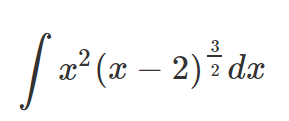Solution: Let F(x) = x   and    F(y) = (x – 2)3/2

Construct the table to solve this problem by tabular integration method

 F(x) Derivative Function F(y) Integration Function (+) x2 (x – 2)3/2 (-)   2x 2/5(x – 2)5/2 (+)   2 4/35(x – 2)7/2 (-)   0 8/315(x – 2)9/2

Use these steps to further solve this integral problem.

1. Multiply F(x) with the first integration of F(y)
2. Multiply the first derivative of F(x) with the second integration of F(y)………and so on.$\int_{}^{} x^{2}(x – 2)^{\frac{3}{2}}dx$ = $\frac{2}{5}x^{2}(x-2)^{\frac{5}{2}} – \frac{8}{35}x(x-2)^{\frac{7}{2}}+ \frac{16}{315}(x-2)^{\frac{9}{2}} + C$  Answer

### Example 8:Solution: Let F(x) = 6x   and    F(y) = cos(2x)

Construct the table to solve this problem by tabular integration

 F(x) Derivative Function F(y) Integration Function (+) 6x3 cos(2x) (-)   18x2 sin(2x)/2 (+)   36x -cos(2x)/4 (-)   36 -sin(2x)/8 0 cos(2x)/16

Use these steps to further solve this integral problem.

1. Multiply F(x) with the first integration of F(y)
2. Multiply the first derivative of F(x) with the second integration of F(y)………and so on.$\int_{}^{} 6x^{3}.cos(2x)dx$ = 3x3sin2x + (9/2)x2cos2x – (9/2)xsin2x – (9/4)cos2x + C   Answer

### Example 9:Solution: Let F(x) = 4x   and    F(y) = e-2x

Construct the table to solve this problem by tabular integration

 F(x) Derivative Function F(y) Integration Function (+) 4x2 e-2x (-)   8x -e-2x/2 (+)   8 e-2x/4 (-)   0 -e-2x/8

Use these steps to further solve this integral problem.

1. Multiply F(x) with the first integration of F(y)
2. Multiply the first derivative of F(x) with the second integration of F(y)………and so on.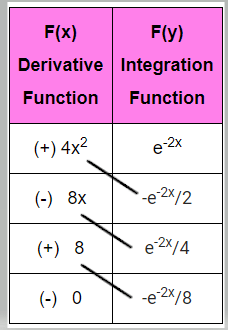$\int_{}^{} 4x^{2}.e^{-2x}dx$ = -2x2e-2x – 2xe-2x – e-2x + C   Answer

## FAQ

### What is the Tabular Integration?

The tabular Integration is also called the DI method to solve integration problems quickly by forming three columns, the first one for “Alternative sign”, the second column for “Derivative function” and the third column for “Integration function”.

### Integration by parts vs Tabular integration Method?

Integration by parts is the traditional method that is used to finds the integral of a product of functions and Tabular integration is a short technique to solve integral problems quickly by letting one of the functions can be differentiated multiple times and the other function can be integrated multiple times with ease.

### When do you can use the tabular integration?

Tabular integration can be used where one function is differentiated until it gets zero, and another function can be integrated simultaneously multiple times. Examples – ∫x6.sinx dx, ∫x3.e4x dx, etc.

Tabular integration can also be used where none of the functions gets zero when differentiated multiple times. Examples – ∫cosx.sinx dx, ∫ex.sin5x dx, etc.

##### Subscribe to Blog via Email

Join 2 other subscribers

Share it...